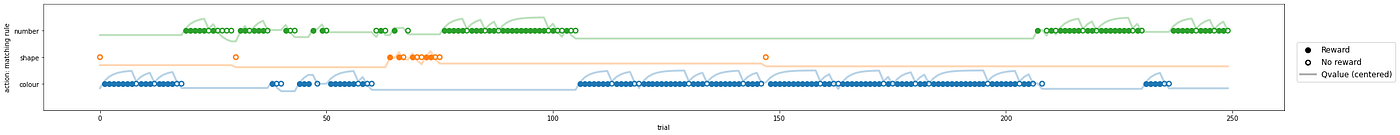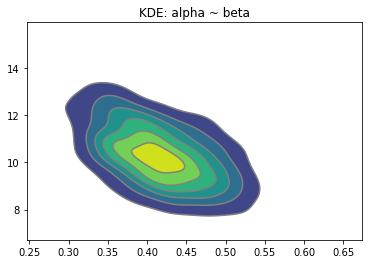# Bayesian Reinforcement Learning & other nonlinear Probabilistic Graphs

Probabilistic programming is a powerful approach that combines Bayesian inference & programmatic logic to model real-world systems. The premise is simple:

1. State Space: Define some (arbitrary) model that encapsulates the mechanics of a system. Arbitrary in that the model can be nonlinear & include any sequence of deterministic & stochastic events.
2. Parameterization: Specify priors over learnable parameters within the state space.
3. Optimization & Diagnostics: Utilized any number of readily available nonlinear optimizers to tune parameters & then perform model diagnostics to assess the fit.

The key contribution is simple:

By using programmatic logic, one can encode the known dynamics of any system. This is extremely flexible. To circumvent the risk of overfitting as a consequence of this flexibility, taking a Bayesian approach allows one to draw on a vast & well-studied body of mathematics to assess model & parameter estimates.

# Reinforcement Learning (RL)

RL is one such example of this model class. In most cases, RL is used as an optimization procedure that generalizes outside of the training domain. One might, however, easily conceptualize how RL could be used as a framework to model human or animal behaviour.

This very approach is taken in our research group, where computational neuroscience relies on RL to represent the cognitive learning processes.

# Model Design

As an illustration, consider a simple RL model:

# Statistical Challenges

The model is conceptually trivial, however, the recursive update equation to Qt(a) makes it a nonlinear system & thus fitting a GML is insufficient. Although Maximum Likelihood estimation may be used directly with any off-the-shelf (scipy.stats) nonlinear optimizer, by taking a Bayesian approach one is afforded the ability to:

1. Learn confidence intervals without the computation of the gradient of the Hessian.
2. Bound search by imposing logical priors.
3. Extend the model to a hierarchical variance structure over multiple participants.

# Probabilistic Programming

Probabilistic programming allows one to write the system as a simple script where a function captures the model as a hypothesized data generating process.

Packages: I use PyMC3, however am equally fond of Pyro. Other good options include STAN, Edward & Tensorflow Probability.

We can generate simulated data to examine the viability of this approach.

## Data Generating Process

We generate data that instantiates our theoretical model & thereafter examine whether or not we are able to recover the true (unknown) parameterization.

We call this function to generate the data & examine the action space distribution.An illustration of the Qt(a) updating process as a function of actions & rewards.

# Model Implementation

Implementing the model is then a simple Python Script (leveraging PyMC3) with basic knowledge of statistical distributions as priors to encode the stochasticity. The important takeaway is the nonlinear functionality in the sequence.

# Results

Easy as that! Now examine the posteriors!Kernel Density Estimation (KDE) between parameter estimates.

# Sensitivity Analysis

As an illustration of how robust this technology is, here I run the computation over a range of alpha & beta values & plot the estimated values against the true value.

Alphas track extremely well, Betas deviate in n, however, this is a byproduct of the systematic entanglement of covariates: large Betas are offset by alpha values. These large Betas also fall outside of the plausible range.

# Conclusion

This approach allows one to specify any arbitrary Bayesian probabilistic graph. Extremely useful in real engineering and other systems that rely on well-understood complex dynamics.

--

--## Zach Wolpe

Statistician, scientist, technologist — writing about stats, data science, math, philosophy, poetry & any other flavours that occupy my mind. Get in touch